# Trigonometry Basics Chapter Exam

Exam Instructions:

Choose your answers to the questions and click 'Next' to see the next set of questions. You can skip questions if you would like and come back to them later with the yellow "Go To First Skipped Question" button. When you have completed the practice exam, a green submit button will appear. Click it to see your results. Good luck!

### Page 1

#### Question 1 1. What's the value of 2sin(θ)cos(θ) given that the equation below is true?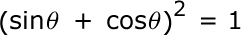#### Question 2 2. What is x?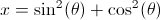#### Question 3 3. What is x?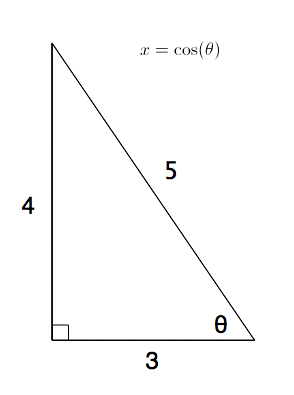#### Question 4 4. What is x?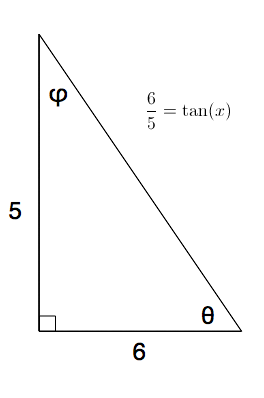#### Question 5 5. What is x?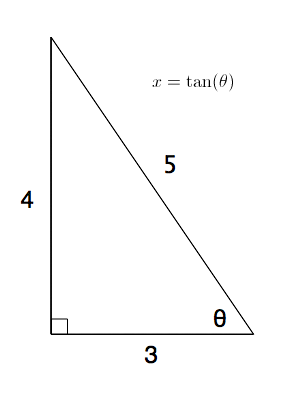### Page 2

#### Question 6 6. Solve the following equation for x.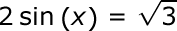#### Question 7 7. What's the value of sec2 (θ) given that the equation below is true?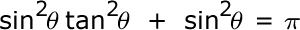#### Question 8 8. What is z in terms of x and y?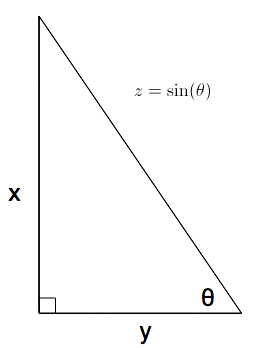#### Question 9 9. Solve for the value of x in the trigonometric equation below. (Use the domain -π/2 to π/2)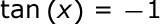#### Question 10 10. Solve the following trigonometric equation for x.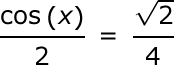### Page 3

#### Question 11 11. Simplify the following expression.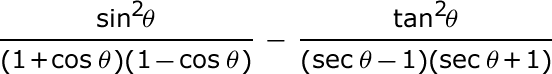#### Question 12 12. Solve the following trigonometric equation for θ.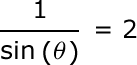#### Question 13 13. What is x?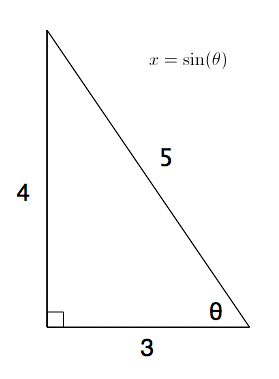#### Question 14 14. Solve the following equation for θ.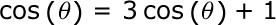#### Question 15 15. Simplify the following expression.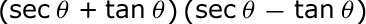### Page 4

#### Question 16 16. What is z in terms of x?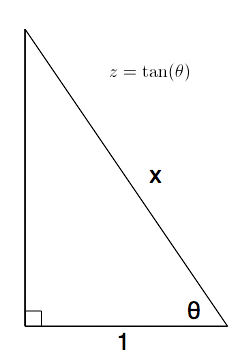#### Question 17 17. What is the value of cos(θ) given that the equation below is true?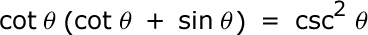#### Question 18 18. The lines a and b are parallel lines of length 5 and 2. The lines d and e are parallel to one another, and both are perpendicular to a and b. What is the length of c?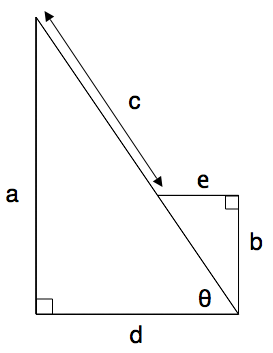#### Question 19 19. What is x?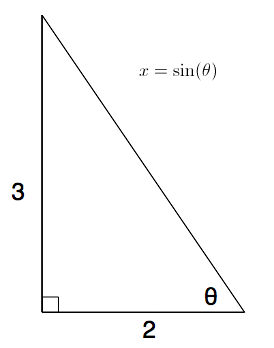#### Trigonometry Basics Chapter Exam Instructions

Choose your answers to the questions and click 'Next' to see the next set of questions. You can skip questions if you would like and come back to them later with the yellow "Go To First Skipped Question" button. When you have completed the practice exam, a green submit button will appear. Click it to see your results. Good luck!

Support